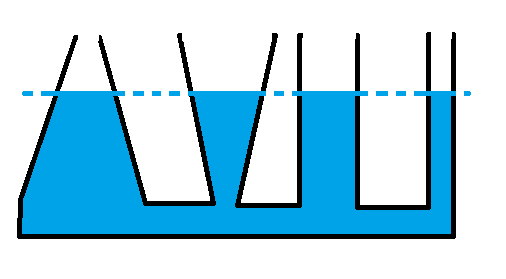homechevron_rightStudychevron_rightPhysics

# Hydrostatic pressure

This online calculator can solve hydrostatic pressure problems by finding unknown value in hydrostatic equation.

This online calculator can solve hydrostatic pressure problems by finding unknown value in hydrostatic equation.
The equation is as follows:

It states that the pressure difference between two elevations in fluid is the product of elevation change, gravity, and density.
Calculator can solve this equation

• for pressure using known density, height and gravity
• for density using known pressure, height and gravity
• for height using known pressure, density and gravity
• for gravity using known pressure, height and density

All formulas are trivial. The input's default values are as follows:

• density is density of water
• gravity is gravity on Earth
• pressure is one atmosphere.

You can also find some theory below the calculator.#### Hydrostatic pressure

Digits after the decimal point: 2
Pressure, Pa

Height, m

Density, kg/m3

Gravity, m/s2

Hydrostatic pressure — pressure difference between two elevations.

The simplified formula, which does not consider, for example, fluid's compression, yet gives good estimations, can be obtained as follows:

The formula depends only on the height of the fluid chamber, and not on its width or length. Given a large enough height, any pressure may be attained. It is believed that this was first demonstrated by Pascal in his barrel experiment.
Pascal's barrel is the name of a hydrostatics experiment allegedly performed by Blaise Pascal in 1646. In the experiment, Pascal inserted a 10-m long (32.8 ft) vertical tube into a barrel filled with water. When water was poured into the vertical tube, Pascal found that the increase in hydrostatic pressure caused the barrel to burst.

This feature of hydrostatics has been called the hydrostatic paradox. As expressed by W. H. Besant,

• Any quantity of liquid, however small, may be made to support any weight, however large.Picture above shows that the static fluid pressure at a given depth does not depend upon the total mass, surface area, or the geometry of the container. It depends only on height of fluid. This is because the pressure to the inclined walls has vertical part, and in left vessel it points up, in middle vessel it points down, thus, adding or removing additional pressure to the bottom pressure, so it is proportional only to the height of fluid.

Sources: